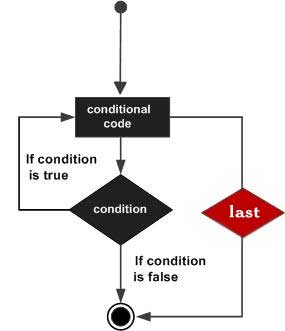# Perl last Statement

When a last statement is encountered inside a loop, the loop is immediately terminated and the program control resumes at the next statement following the loop. You can provide a LABEL with last statement where LABEL is the label for a loop. A last statement can be used inside a nested loop where it will be applicable to the nearest loop if a LABEL is not specified.

If there is any continue block on the loop, then it is not executed. You will see the continue statement in a separate chapter.

## Syntax

The syntax of a last statement in Perl is −

```last [LABEL];
```

## Flow Diagram## Example 1

```#!/usr/local/bin/perl

\$a = 10;
while( \$a < 20 ) {
if( \$a == 15) {
# terminate the loop.
\$a = \$a + 1;
last;
}
print "value of a: \$a\n";
\$a = \$a + 1;
}
```

When the above code is executed, it produces the following result −

```value of a: 10
value of a: 11
value of a: 12
value of a: 13
value of a: 14
```

## Example 2

Let's take one example where we are going to use a LABEL along with next statement −

```#!/usr/local/bin/perl

\$a = 0;
OUTER: while( \$a < 4 ) {
\$b = 0;
print "value of a: \$a\n";
INNER:while ( \$b < 4) {
if( \$a == 2) {
# terminate outer loop
last OUTER;
}
\$b = \$b + 1;
print "Value of b : \$b\n";
}
print "\n";
\$a = \$a + 1;
}
```

When the above code is executed, it produces the following result −

```value of a : 0
Value of b : 1
Value of b : 2
Value of b : 3
Value of b : 4

value of a : 1
Value of b : 1
Value of b : 2
Value of b : 3
Value of b : 4

value of a : 2
```
perl_loops.htm